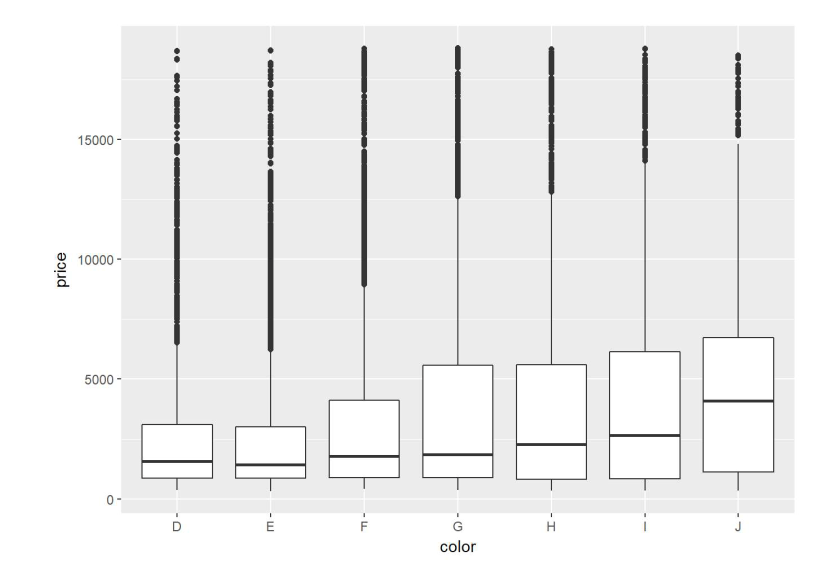# R Tutorial 8: Data Visualisation

## Why do we need visualisation?

Firstly, it helps us identify areas that require attention easily, and secondly, any nice looking data usually serve to assist in understand and convince the readers.

Only some examples will be covered here, feel free to explore the functionality of ggplot here in this neat cheatsheet: https://www.rstudio.com/wp-content/uploads/2015/03/ggplot2-cheatsheet.pdf

Charts that we are going to cover in this tutorial:

1. Single Variable Bar Chart
2. Single Variable Density Plot
3. Categorical Variables Bar Charts
4. Box Plots

In this tutorial, we utilise the package ggplot2 and use the same dataset diamonds.

```library(ggplot2)
library(dplyr)
```

## 1. Single Variable Bar Chart

You will tell R to look for data from the dataframe “diamonds” using ggplot(), before adding other details to the plotting such as the type of plot using the geom_bar() (or other types of plots, which you will see below). After which, depending on the different type of plots, the aes() function usually will be used to define which are the variables to be used for the plotting.

```ggplot(diamonds) + geom_bar(aes(x=cut,fill=cut))
```## 2. Single Variable Density Plot

So here, beside the type of plot, we can include the title “Distribution of Price” into the visualisation.

```ggplot(diamonds) + geom_density(aes(x=price))+ggtitle("Distribution of Prices")
```## 3. Categorical Variables Bar Charts

In this plot, we add another function call facet_wrap(~cut), which ask R to divide the data set to plot the clarity of different cuts, so you don’t have to plot multiple times.

```ggplot(diamonds) + geom_bar(aes(x=clarity, fill=clarity))+facet_wrap(~cut)
```## 4. Box Plot

Combining pipe operator from dplyr, note that as we don’t have to specify that the data is from diamonds as it take the data after the filter.

```diamonds %>%
filter(cut=="Ideal")%>%
ggplot(aes(x=color,y=price))+
geom_boxplot()
```